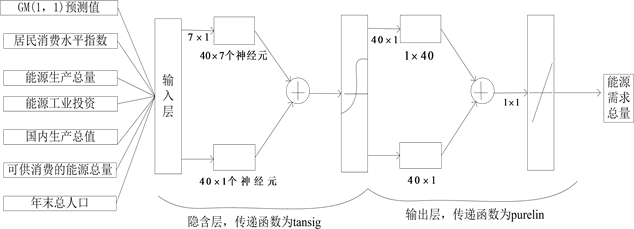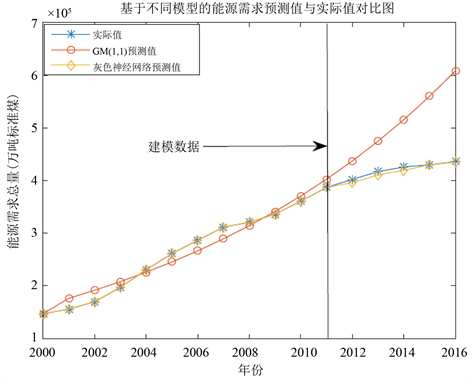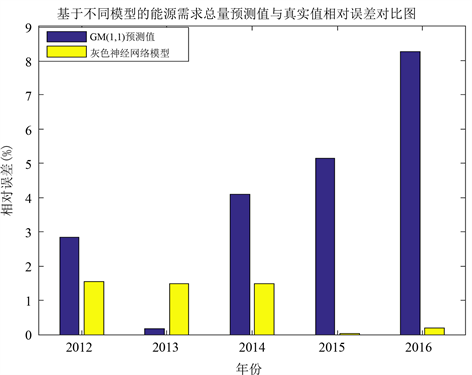﻿ 灰色神经网络组合模型在能源需求中的应用

# 灰色神经网络组合模型在能源需求中的应用Application of the Gray Neural Network Combination Model in Forecasting Energy Demand of China

Abstract: Energy is the cornerstone of social development. With the development of economy, the demand for energy is increasing year by year. So it is very important to forecast the energy demand with high precision. In this paper, based on GM(1, 1) model and BP neural network model, a tandem type grey neural network model is established to forecast the national total energy demand using the resident consumption level index, the total energy production, the energy industry investment, the gross domestic product, the total energy available for consumption, and the total population at year-end as the influence indexes. The actual data of total energy consumption in 2000~2011 were selected as modeling data, and those in 2012~2016 were selected as testing data. Comparative analysis of GM(1, 1) and gray neural network prediction accuracy was made. The results show that the grey neural network model has the highest prediction accuracy and the mean variance of the prediction error is 0.5840, which is less than the mean variance of the single GM(1, 1) prediction error.

1. 引言

2. 研究方法

2.1. GM(1, 1)预测模型

1982年，邓聚龙教授首次提出了灰色理论(Grey system)  ，该理论对原始数作累加生成的的近似指数规律再进行建模的方法。灰色模型有严格的理论基础，最大的优点是所需数据少，一般只需要4个数据就能对未来数据进行预测，为此该理论被广泛应用于各个领域。能源需求量既有已知的信息，也有未确定的信息，可看做一个灰色系统。GM(1, 1)灰色模型  建立如下：

${x}^{\left(1\right)}\left(k\right)=\underset{i=1}{\overset{k}{\sum }}{x}^{\left(0\right)}\left(i\right)\left(k=1,2,\cdots ,n\right)$ . (1)

${Z}^{\left(1\right)}$${X}^{\left(1\right)}$ 的紧邻均值生成序列： ${Z}^{\left(1\right)}=\left({z}^{\left(1\right)}\left(2\right),{z}^{\left(1\right)}\left(3\right),\cdots ,{z}^{\left(1\right)}\left(n\right)\right)$ ，其中：

${z}^{\left(1\right)}\left(k\right)=0.5\left({x}^{\left(1\right)}\left(k\right)+0.5{x}^{\left(1\right)}\left(k-1\right)\right),\text{\hspace{0.17em}}k=2,3,\cdots ,n$ . (2)

${x}^{\left(0\right)}\left(k\right)+a{z}^{\left(1\right)}\left(k\right)=b,\text{\hspace{0.17em}}k=2,3,\cdots ,n$ . (3)

$u={\left[a,b\right]}^{\text{T}}$ 为参数列，且有

$B=\left[\begin{array}{cc}-{z}^{\left(1\right)}\left(2\right)& 1\\ -{z}^{\left(1\right)}\left(3\right)& 1\\ ⋮& ⋮\\ -{z}^{\left(1\right)}\left(n\right)& 1\end{array}\right]$ , $Y=\left[\begin{array}{l}{x}^{\left(0\right)}\left(2\right)\\ {x}^{\left(0\right)}\left(3\right)\\ ⋮\\ {x}^{\left(0\right)}\left(n\right)\end{array}\right]$(4)

$\stackrel{^}{u}={\left({B}^{\text{T}}B\right)}^{-1}{B}^{\text{T}}Y$ (5)

$B,Y,\stackrel{^}{u}$ 满足式(4)和式(5)，相应的白化微分方程为

$\frac{\text{d}{x}^{\left(1\right)}}{\text{d}t}+a{x}^{\left(1\right)}\left(t\right)=b$ . (6)

GM(1, 1)模型的时间序列为：

${\stackrel{^}{x}}^{\left(1\right)}\left(k+1\right)=\left({x}^{\left(0\right)}\left(1\right)-\frac{b}{a}\right){\text{e}}^{-ak}+\frac{b}{a},\text{\hspace{0.17em}}k=1,2,\cdots ,n$ (7)

${\stackrel{^}{x}}^{\left(1\right)}\left(k+1\right)={\stackrel{^}{x}}^{\left(1\right)}\left(k+1\right)-{\stackrel{^}{x}}^{\left(1\right)}\left(k\right)=\left(1-{\text{e}}^{a}\right)\left({\stackrel{^}{x}}^{\left(0\right)}\left(1\right)-\frac{b}{a}\right){\text{e}}^{-ak}$ (8)

$\epsilon \left(k\right)=\frac{{x}^{\left(0\right)}\left(k\right)-{\stackrel{^}{x}}^{\left(0\right)}\left(k\right)}{{x}^{\left(0\right)}\left(k\right)}$ (9)

2.2. BP神经网络模型

1985年，美国加州大学的鲁梅尔哈特和麦克莱兰等人提出BP神经网络  ，神经网络已广泛应用于各个领域。从结构上看，该模型具有输入层，隐含层和输出层，是一种典型的多层前向型神经网络。该网络广泛应用于图像处理、模式识别、语言处理、预测分析等领域。

1) 神经元模型

$Y=f\left(wp+b\right)$ (10)

2) 激励函数

$f\left(x\right)=\frac{1}{1+{\text{e}}^{-cx}}$ (11)

3) BP神经网络的学习算法

BP神经网络的误差反向传播算法基本思想是对一定数量的样本对输入和期望输出进行学习，将输入数据传送至各个神经元，经隐含层和输出层计算后，输出层输出对应的预测值。如果预测值与实际值误差较大时，输出层进行反向传播该误差，进行权值和阈值的调整，不断修正误差，直至满足精度要求。其算法核心的思想是：权值和阈值的调整总是沿着误差函数最快的方向，即负梯度方向。

${W}_{1}={\left({w}_{ij}^{1}\right)}_{l×m},\text{\hspace{0.17em}}\text{\hspace{0.17em}}\text{\hspace{0.17em}}i=1,2,\cdots ,l,\text{\hspace{0.17em}}j=1,2,\cdots ,m$ (12)

${W}_{2}={\left({w}_{ij}^{2}\right)}_{n×l},\text{\hspace{0.17em}}\text{\hspace{0.17em}}\text{\hspace{0.17em}}\text{\hspace{0.17em}}i=1,2,\cdots ,n,\text{\hspace{0.17em}}j=1,2,\cdots ,l$ (13)

${O}_{j}=f\left(\underset{i=1}{\overset{m}{\sum }}{w}_{ji}^{2}{x}_{i}-{\theta }_{l}^{1}\right)=f\left(ne{t}_{j}\right),\text{\hspace{0.17em}}j=1,2,\cdots ,l$ (14)

${z}_{k}=g\left(\underset{j=1}{\overset{l}{\sum }}{w}_{kj}^{2}{O}_{j}-{\theta }_{k}^{2}\right)=g\left(ne{t}_{k}\right),\text{\hspace{0.17em}}k=1,2,\cdots ,n$ (15)

$E=\frac{1}{2}\underset{k=1}{\overset{n}{\sum }}{\left({y}_{k}-{z}_{k}\right)}^{2}$ (16)

$\Delta {w}_{ji}^{1}=-\eta \frac{\partial E}{\partial {w}_{ji}^{1}}$ , $\Delta {w}_{kj}^{2}=-\eta \frac{\partial E}{\partial {w}_{kj}^{2}}$ (17)

${R}^{2}=\frac{{\left(l\underset{i=0}{\overset{l}{\sum }}{\stackrel{^}{y}}_{i}{y}_{i}-\underset{i=0}{\overset{l}{\sum }}{\stackrel{^}{y}}_{i}\underset{i=0}{\overset{l}{\sum }}{y}_{i}\right)}^{2}}{\left(l\underset{i=0}{\overset{l}{\sum }}{\stackrel{^}{y}}_{i}^{2}-{\left(\underset{i=0}{\overset{l}{\sum }}{\stackrel{^}{y}}_{i}^{2}\right)}^{2}\right)\left(l\underset{i=0}{\overset{l}{\sum }}{y}_{i}^{2}-{\left(\underset{i=0}{\overset{l}{\sum }}{y}_{i}^{2}\right)}^{2}\right)}$ , (18)

2.3. 组合灰色神经网络模型

$\delta =\sqrt{\underset{k=1}{\overset{n}{\sum }}{\left({x}^{\left(0\right)}\left(k\right)-{\stackrel{^}{x}}^{\left(0\right)}\left(k\right)\right)}^{2}/n}$ (19)

3. 能源需求实例分析

3.1. 数据收集Figure 1. Gray network structure diagram

3.2. 灰色GM(1, 1)预测模型

${\stackrel{^}{x}}^{\left(1\right)}\left(k+1\right)=2048563.152{\text{e}}^{0.0825k}-1901599.152,\text{\hspace{0.17em}}k=1,2,\cdots ,n$Table 2. Comparison of forecast and actual value of total energy demand in 2012~2016 based on different models

3.3. 组合灰色神经网络预测模型

1) 模型的输入、输出参数的选择

2) 模型的结构设计

3) 模型网络参数的选取及参数设定

3.4. 模型精度比较

4. 结论Figure 2. Gray neural network topologyFigure 3. Energy demand forecast based on different models versus real value chartFigure 4. Comparison chart of relative error between predicted and actual value of energy demand based on different models

1) 由于全国能源需求总量具有波动性和非线性的特点，建立了灰色GM(1, 1)预测模型和灰色神经网络模型，并以2000~2011年的能源需求量基础数据作为训练样本，以2012~2016年的数据为检验样本，经计算预测值与真实值的误差均在13%以内，表明建立的模型是有效的。

2) 以全国能源需求为例分别利用GM(1, 1)和灰色神经网络对能源需求进行预测，其预测误差的均方差分别为：12.3462，0.5840，可以看出灰色神经网络对能源需求总量预测的精度最高。

3) 灰色神经网络模型具有更好的预测效果，能够弥补单一模型的不足。

 周扬, 吴文祥, 胡莹, 等. 基于组合模型的能源需求预测[J]. 中国人口•资源与环境, 2010, 20(4): 63-68.

 索瑞霞, 王福林. 组合预测模型在能源消费预测中的应用[J]. 数学的实践与认识, 2010, 40(18): 80-85.

 张帆. 基于GM-GRNN的能源需求预测模型研究[J]. 湖北汽车工业学院学报, 2012, 26(3): 65-69.

 崔吉峰, 乞建勋, 杨尚东. 基于粒子群改进BP神经网络的组合预测模型及其应用[J]. 中南大学学报(自然科学版), 2009, 40(1): 190-194.

 赵荣. 云南省某州(市)能源预测模型的研究[D]: [硕士学位论文]. 昆明: 昆明理工大学, 2017.

 虞亚平. 京津冀能源需求预测分析及发展对策研究[D]: [硕士学位论文]. 天津: 天津理工大学, 2017.

 邓聚龙. 灰色系统基本方法[M]. 武汉: 华中工学院出版社, 1987: 96-108.

 李剑波, 鲜学福. 基于灰色神经网络模型的重庆能源需求预测[J]. 西南大学学报(自然科学版), 2016, 38(6): 136-141.

 李柏年, 吴礼斌. MATLAB数据分析方法[M]. 北京: 机械工业出版社, 2012: 170-172.

 中国省统计局. 中国统计年鉴(2017) [M]. 北京: 中国统计出版社, 2017.

Top